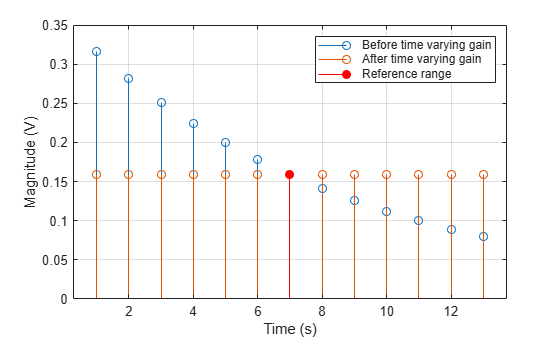# step

System object: phased.TimeVaryingGain
Package: phased

Apply time varying gains to input signal

## Syntax

```Y = step(H,X) Y = step(H,X,L) ```

## Description

Note

Starting in R2016b, instead of using the `step` method to perform the operation defined by the System object™, you can call the object with arguments, as if it were a function. For example, ```y = step(obj,x)``` and `y = obj(x)` perform equivalent operations.

`Y = step(H,X)` applies time varying gains to the input signal matrix `X`. The process equalizes power levels across all samples to match a given reference range. The compensated signal is returned in `Y`. `X` can be a column vector, a matrix, or a cube. The gain is applied to each column in `X` independently. The number of rows in `X` cannot exceed the length of the loss vector specified in the `RangeLoss` property. `Y` has the same dimensionality as `X`. `X` can be single or double precision.

The size of the first dimension of the input matrix can vary to simulate a changing signal length. A size change can occur, for example, in the case of a pulse waveform with variable pulse repetition frequency.

`Y = step(H,X,L)` in addition, specifies the range loss, `L` as a columns vector. Use this argument only when you set the `RangeLossSource` property to `'Input port'`. The length of `L` must be equal to or greater than the number of rows of `X`. `L` can be single or double precision.

Note

The object performs an initialization the first time the object is executed. This initialization locks nontunable properties and input specifications, such as dimensions, complexity, and data type of the input data. If you change a nontunable property or an input specification, the System object issues an error. To change nontunable properties or inputs, you must first call the `release` method to unlock the object.

## Examples

expand all

Apply time varying gain to a signal to compensate for signal power loss due to range.

First, create a signal with range loss. Set the reference loss to 16 dB.

```rngloss = 10:22; refloss = 16; t = (1:length(rngloss))'; x = 1./db2mag(rngloss(:));```

Then add gain to compensate for range loss.

```gain = phased.TimeVaryingGain('RangeLoss',rngloss,'ReferenceLoss',refloss); y = gain(x);```

Plot the signal with loss and the compensated signal.

```tref = find(rngloss==refloss); stem([t t],[abs(x) abs(y)]) hold on stem(tref,x(tref),'filled','r') xlabel('Time (s)'); ylabel('Magnitude (V)') grid on legend('Before time varying gain','After time varying gain',... 'Reference range')```